Momentum and Its Conservation - Lesson 2 - The Law of Momentum Conservation

# Momentum Conservation Principle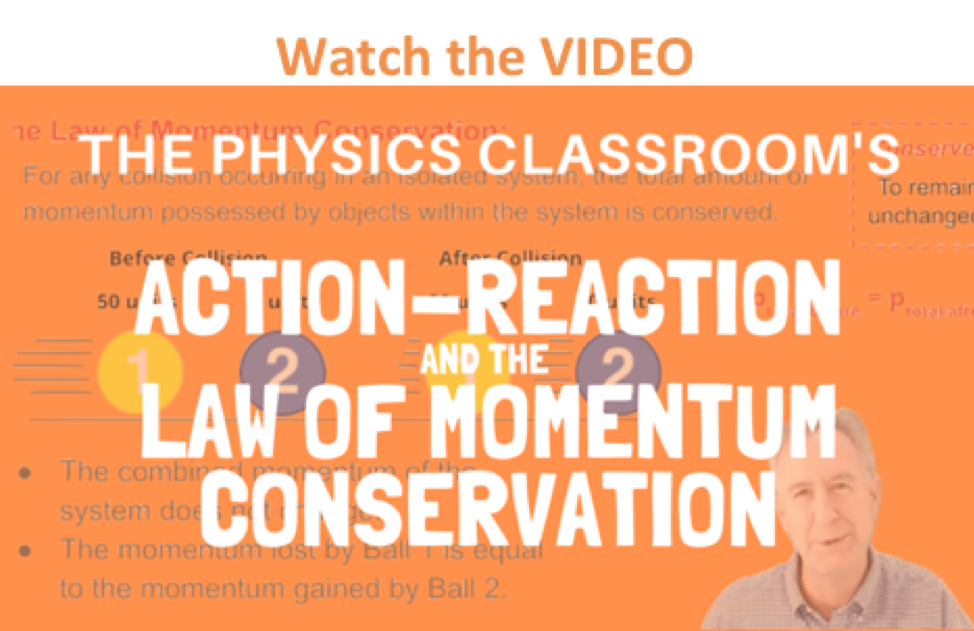One of the most powerful laws in physics is the law of momentum conservation. The law of momentum conservation can be stated as follows.

For a collision occurring between object 1 and object 2 in an isolated system, the total momentum of the two objects before the collision is equal to the total momentum of the two objects after the collision. That is, the momentum lost by object 1 is equal to the momentum gained by object 2.

The above statement tells us that the total momentum of a collection of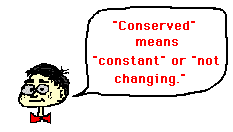objects (a system) is conserved - that is, the total amount of momentum is a constant or unchanging value. This law of momentum conservation will be the focus of the remainder of Lesson 2. To understand the basis of momentum conservation, let's begin with a short logical proof.

### The Logic Behind Momentum Conservation

Consider a collision between two objects - object 1 and object 2. For such a collision, the forces acting between the two objects are equal in magnitude and opposite in direction (Newton's third law). This statement can be expressed in equation form as follows.The forces act between the two objects for a given amount of time. In some cases, the time is long; in other cases the time is short. Regardless of how long the time is, it can be said that the time that the force acts upon object 1 is equal to the time that the force acts upon object 2. This is merely logical. Forces result from interactions (or contact) between two objects. If object 1 contacts object 2 for 0.050 seconds, then object 2 must be contacting object 1 for the same amount of time (0.050 seconds). As an equation, this can be stated as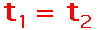Since the forces between the two objects are equal in magnitude and opposite in direction, and since the times for which these forces act are equal in magnitude, it follows that the impulses experienced by the two objects are also equal in magnitude and opposite in direction. As an equation, this can be stated as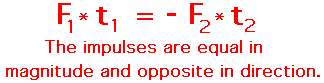But the impulse experienced by an object is equal to the change in momentum of that object (the impulse-momentum change theorem). Thus, since each object experiences equal and opposite impulses, it follows logically that they must also experience equal and opposite momentum changes. As an equation, this can be stated as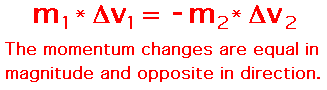###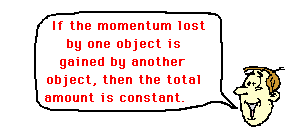The Law of Momentum Conservation

The above equation is one statement of the law of momentum conservation. In a collision, the momentum change of object 1 is equal to and opposite of the momentum change of object 2. That is, the momentum lost by object 1 is equal to the momentum gained by object 2. In most collisions between two objects, one object slows down and loses momentum while the other object speeds up and gains momentum. If object 1 loses 75 units of momentum, then object 2 gains 75 units of momentum. Yet, the total momentum of the two objects (object 1 plus object 2) is the same before the collision as it is after the collision. The total momentum of the system (the collection of two objects) is conserved.

A useful analogy for understanding momentum conservation involves a money transaction between two people. Let's refer to the two people as Jack and Jill. Suppose that we were to check the pockets of Jack and Jill before and after the money transaction in order to determine the amount of money that each possesses. Prior to the transaction, Jack possesses \$100 and Jill possesses \$100. The total amount of money of the two people before the transaction is \$200. During the transaction, Jack pays Jill \$50 for the given item being bought. There is a transfer of \$50 from Jack's pocket to Jill's pocket. Jack has lost \$50 and Jill has gained \$50. The money lost by Jack is equal to the money gained by Jill. After the transaction, Jack now has \$50 in his pocket and Jill has \$150 in her pocket. Yet, the total amount of money of the two people after the transaction is \$200. The total amount of money (Jack's money plus Jill's money) before the transaction is equal to the total amount of money after the transaction. It could be said that the total amount of money of the system (the collection of two people) is conserved. It is the same before as it is after the transaction.

A useful means of depicting the transfer and the conservation of money between Jack and Jill is by means of a table.The table shows the amount of money possessed by the two individuals before and after the interaction. It also shows the total amount of money before and after the interaction. Note that the total amount of money (\$200) is the same before and after the interaction - it is conserved. Finally, the table shows the change in the amount of money possessed by the two individuals. Note that the change in Jack's money account (-\$50) is equal to and opposite of the change in Jill's money account (+\$50).

For any collision occurring in an isolated system, momentum is conserved. The total amount of momentum of the collection of objects in the system is the same before the collision as after the collision. A common physics lab involves the dropping of a brick upon a cart in motion.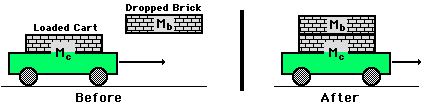The dropped brick is at rest and begins with zero momentum. The loaded cart (a cart with a brick on it) is in motion with considerable momentum. The actual momentum of the loaded cart can be determined using the velocity (often determined by a ticker tape analysis) and the mass. The total amount of momentum is the sum of the dropped brick's momentum (0 units) and the loaded cart's momentum. After the collision, the momenta of the two separate objects (dropped brick and loaded cart) can be determined from their measured mass and their velocity (often found from a ticker tape analysis). If momentum is conserved during the collision, then the sum of the dropped brick's and loaded cart's momentum after the collision should be the same as before the collision. The momentum lost by the loaded cart should equal (or approximately equal) the momentum gained by the dropped brick. Momentum data for the interaction between the dropped brick and the loaded cart could be depicted in a table similar to the money table above.

 Before Collision  Momentum After Collision Momentum Change in Momentum Dropped Brick 0 units 14 units +14 units Loaded Cart 45 units 31 units -14 units Total 45 units 45 units

Note that the loaded cart lost 14 units of momentum and the dropped brick gained 14 units of momentum. Note also that the total momentum of the system (45 units) was the same before the collision as it was after the collision.Collisions commonly occur in contact sports (such as football) and racket and bat sports (such as baseball, golf, tennis, etc.). Consider a collision in football between a fullback and a linebacker during a goal-line stand. The fullback plunges across the goal line and collides in midair with the linebacker. The linebacker and fullback hold each other and travel together after the collision. The fullback possesses a momentum of 100 kg*m/s, East before the collision and the linebacker possesses a momentum of 120 kg*m/s, West before the collision. The total momentum of the system before the collision is 20 kg*m/s, West (review the section on adding vectors if necessary). Therefore, the total momentum of the system after the collision must also be 20 kg*m/s, West. The fullback and the linebacker move together as a single unit after the collision with a combined momentum of 20 kg*m/s. Momentum is conserved in the collision. A vector diagram can be used to represent this principle of momentum conservation; such a diagram uses an arrow to represent the magnitude and direction of the momentum vector for the individual objects before the collision and the combined momentum after the collision.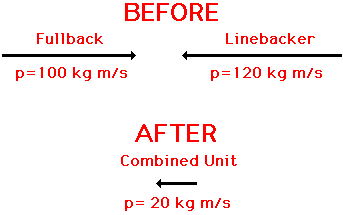Now suppose that a medicine ball is thrown to a clown who is at rest upon the ice; the clown catches the medicine ball and glides together with the ball across the ice. The momentum of the medicine ball is 80 kg*m/s before the collision. The momentum of the clown is 0 m/s before the collision. The total momentum of the system before the collision is 80 kg*m/s. Therefore, the total momentum of the system after the collision must also be 80 kg*m/s. The clown and the medicine ball move together as a single unit after the collision with a combined momentum of 80 kg*m/s. Momentum is conserved in the collision.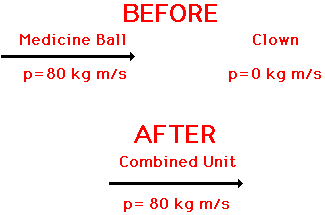Momentum is conserved for any interaction between two objects occurring in an isolated system. This conservation of momentum can be observed by a total system momentum analysis or by a momentum change analysis. Useful means of representing such analyses include a momentum table and a vector diagram. Later in Lesson 2, we will use the momentum conservation principle to solve problems in which the after-collision velocity of objects is predicted.

### Watch It!

Using motion detectors and carts on a low-friction track, one can collect data to demonstrate the law of conservation of momentum. The video below demonstrates the process.

### We Would Like to Suggest ...Sometimes it isn't enough to just read about it. You have to interact with it! And that's exactly what you do when you use one of The Physics Classroom's Interactives. We would like to suggest that you combine the reading of this page with the use of our Cart and Brick Interactive, Exploding Carts Interactive, and/or our Collision Carts Ineractive. These Interactives can be found in the Physics Interactive section of our website and provide an interactive experience in analyzing the momentum of individual objects and systems of objects in collisions.

Express your understanding of the concept and mathematics of momentum by answering the following questions. Click on the button to view the answers.

1. When fighting fires, a firefighter must use great caution to hold a hose that emits large amounts of water at high speeds. Why would such a task be difficult?

2. A large truck and a Volkswagen have a head-on collision.

a. Which vehicle experiences the greatest force of impact?

b. Which vehicle experiences the greatest impulse?

c. Which vehicle experiences the greatest momentum change?

d. Which vehicle experiences the greatest acceleration?

3. Miles Tugo and Ben Travlun are riding in a bus at highway speed on a nice summer day when an unlucky bug splatters onto the windshield. Miles and Ben begin discussing the physics of the situation. Miles suggests that the momentum change of the bug is much greater than that of the bus. After all, argues Miles, there was no noticeable change in the speed of the bus compared to the obvious change in the speed of the bug. Ben disagrees entirely, arguing that that both bug and bus encounter the same force, momentum change, and impulse. Who do you agree with? Support your answer.

4. If a ball is projected upward from the ground with ten units of momentum, what is the momentum of recoil of the Earth? ____________ Do we feel this? Explain.

5. If a 5-kg bowling ball is projected upward with a velocity of 2.0 m/s, then what is the recoil velocity of the Earth (mass = 6.0 x 1024 kg).

6. A 120 kg lineman moving west at 2 m/s tackles an 80 kg football fullback moving east at 8 m/s. After the collision, both players move east at 2 m/s. Draw a vector diagram in which the before- and after-collision momenta of each player is represented by a momentum vector. Label the magnitude of each momentum vector.

7. In an effort to exact the most severe capital punishment upon a rather unpopular prisoner, the execution team at the Dark Ages Penitentiary search for a bullet that is ten times as massive as the rifle itself. What type of individual would want to fire a rifle that holds a bullet that is ten times more massive than the rifle? Explain.

8. A baseball player holds a bat loosely and bunts a ball. Express your understanding of momentum conservation by filling in the tables below.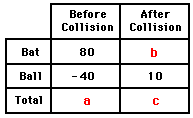9. A Tomahawk cruise missile is launched from the barrel of a mobile missile launcher. Neglect friction. Express your understanding of momentum conservation by filling in the tables below.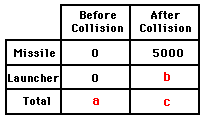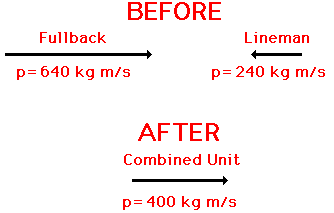Next Section: